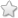# TNSB - 8 - Maths :: Geometry - Term 2

## Course Content / Subtopic list:

1. The Pythagorean Theorem - Statement Of The Theorem, Visual Illustration, Proof Of The Theorem Using Similarity Of Triangles
2. Converse Of Pythagoras Theorem - Pythagorean Triplets, Use Of Pythagoras Theorem In Problems.
3. The Altitude –On –Hypotenuse Theorem (Without Proof) - Theorem, Examples
4. Construction Of Trapeziums
5. Construction Of Parallelograms
0.00(0 votes)